# Various examples in Basis Path Testing

Prerequisite – Basis Path Testing
We have seen the steps involved in designing the test cases for a program using the basis path testing in the previous article. Now, let’s solve an example following the same steps.

Question : Consider the given program that checks if a number is prime or not. For the following program :

1. Draw the Control Flow Graph
2. Calculate the Cyclomatic complexity using all the methods
3. List all the Independent Paths
4. Design test cases from independent paths

 `int` `main() ` `{ ` `    ``int` `n, index; ` `    ``cout << ``"Enter a number: "` `<< endl; ` `    ``cin >> n; ` `    ``index = 2; ` `    ``while` `(index <= n - 1) { ` `        ``if` `(n % index == 0) { ` `            ``cout << ``"It is not a prime number"` `<< endl; ` `            ``break``; ` `        ``} ` `        ``index++; ` `    ``} ` `    ``if` `(index == n) ` `        ``cout << ``"It is a prime number"` `<< endl; ` `} ``// end main `

Solution :
1. Draw the Control Flow Graph –

• Step-1:
Start numbering the statements after declaration of the variables (if no variables have been initialized in that statement). However, if a variable has been initialized and declared in the same line, then numbering should start from that line itself.

For the given program, this is how numbering will be done:

```int main()
{
int n, index;
1    cout << "Enter a number: " <> n;
3    index = 2;
4    while (index <= n - 1)
5    {
6        if (n % index == 0)
7        {
8            cout << "It is not a prime number" << endl;
9            break;
10        }
11        index++;
12    }
13    if (index == n)
14        cout << "It is a prime number" << endl;
15  } // end main
```
• Step-2:
Put the sequential statements into one single node. For example, statements 1, 2 and 3 are all sequential statements and hence should be combined into a single node. And for other statements, we will follow the notations as discussed here.

Note –
Use alphabetical numbering on nodes for simplicity.

The graph obtained will be as follows :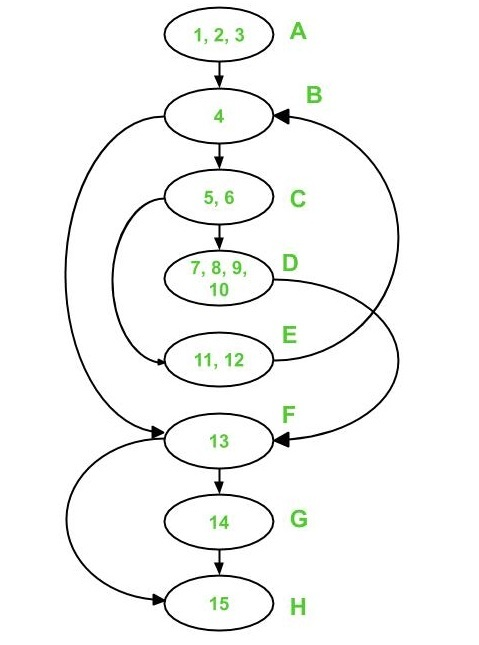2. Calculate the Cyclomatic complexity :

• Method-1:
`V(G) = e - n + 2*p `

In the above control flow graph,

```where, e = 10, n = 8 and p = 1

Therefore,
Cyclomatic Complexity V(G)
= 10 - 8 + 2 * 1
= 4
```
• Method-2:
`V(G) = d + p `

In the above control flow graph,

```where, d = 3 (Node B, C and F) and p = 1

Therefore,
Cyclomatic Complexity V(G)
= 3 + 1
= 4
```
• Method-3:
`V(G) = Number of Regions `

In the above control flow graph, there are 4 regions as shown below :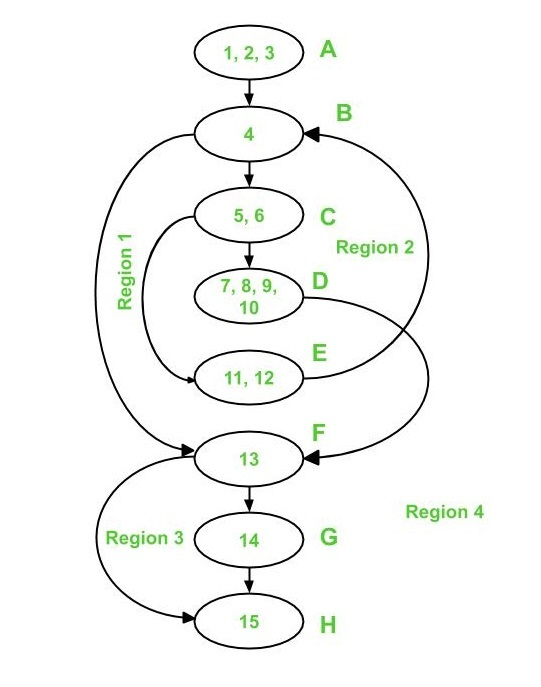```Therefore, there are 4 regions: R1, R2, R3 and R4

Cyclomatic Complexity V(G)
= 1 + 1 + 1 + 1
= 4
```

It is important to note that all three methods give same value for cyclomatic complexity V(G).

3. Independent Paths :
As the cyclomatic complexity V(G) for the graph has come out to be 4, therefore there are 4 independent paths.
Edges covered (marked with red) by Path 1 are:

```Path 1 : A - B - F - G - H
```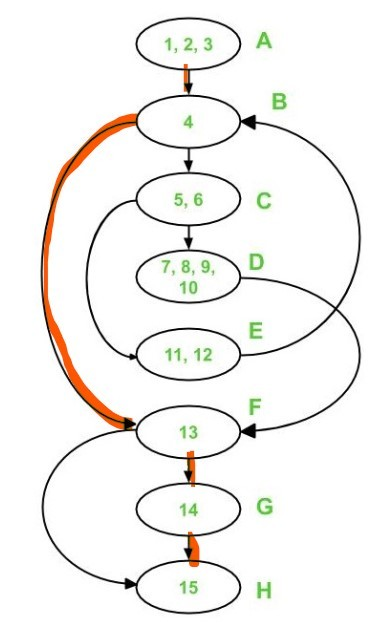Edges covered by Path 1 and Path 2 are shown below :

```Path 2 : A - B - F - H
```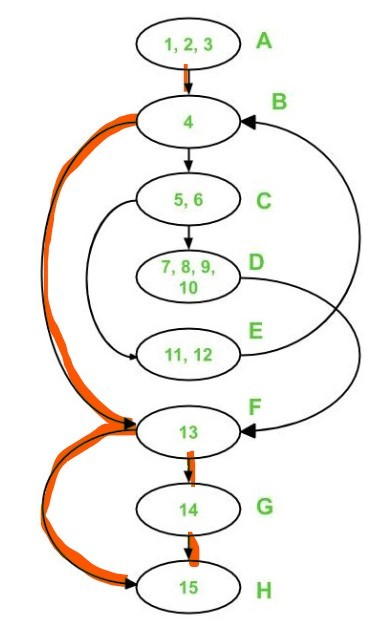Edges covered by Path 1, Path 2 and Path 3 are :

```Path 3 : A - B - C - E - B - F - G - H
```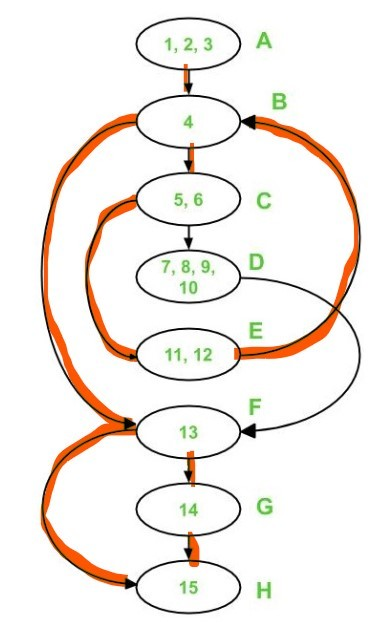Now only 2 edges are left uncovered i.e. edge C-D and edge D-F. Hence, Path 4 must include these two edges.

```Path 4 : A - B - C - D - F - H
```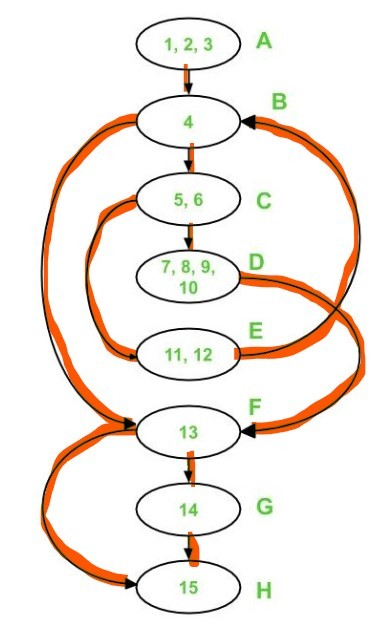Each of these paths have introduced at least one new edge which has not been traversed before.

Note –
Independent paths are not necessarily unique.

4. Test cases :
To derive test cases, we have to use the independent paths obtained previously. To design a test case, provide input to the program such that each independent path is executed.
For the given program, the following test cases will be obtained:

Test case ID Input Number Output Independent Path covered
1 1 No output A-B-F-H
2 2 It is a prime number A-B-F-G-H
3 3 It is a prime number A-B-C-E-B-F-G-H
4 4 It is not a prime number A-B-C-D-F-H

Attention reader! Don’t stop learning now. Get hold of all the important CS Theory concepts for SDE interviews with the CS Theory Course at a student-friendly price and become industry ready.

My Personal Notes arrow_drop_upCheck out this Author's contributed articles.

If you like GeeksforGeeks and would like to contribute, you can also write an article using contribute.geeksforgeeks.org or mail your article to contribute@geeksforgeeks.org. See your article appearing on the GeeksforGeeks main page and help other Geeks.

Please Improve this article if you find anything incorrect by clicking on the "Improve Article" button below.

Article Tags :

Be the First to upvote.

Please write to us at contribute@geeksforgeeks.org to report any issue with the above content.